# Regulated Power Sources

## Discrete Semiconductor Devices and Circuits

• #### Question 1

 Don’t just sit there! Build something!!

Learning to mathematically analyze circuits requires much study and practice. Typically, students practice by working through lots of sample problems and checking their answers against those provided by the textbook or the instructor. While this is good, there is a much better way.

You will learn much more by actually building and analyzing real circuits, letting your test equipment provide the “answers” instead of a book or another person. For successful circuit-building exercises, follow these steps:

1. Carefully measure and record all component values prior to circuit construction, choosing resistor values high enough to make damage to any active components unlikely.
2. Draw the schematic diagram for the circuit to be analyzed.
3. Carefully build this circuit on a breadboard or other convenient medium.
4. Check the accuracy of the circuit’s construction, following each wire to each connection point, and verifying these elements one-by-one on the diagram.
5. Mathematically analyze the circuit, solving for all voltage and current values.
6. Carefully measure all voltages and currents, to verify the accuracy of your analysis.
7. If there are any substantial errors (greater than a few percent), carefully check your circuit’s construction against the diagram, then carefully re-calculate the values and re-measure.

When students are first learning about semiconductor devices, and are most likely to damage them by making improper connections in their circuits, I recommend they experiment with large, high-wattage components (1N4001 rectifying diodes, TO-220 or TO-3 case power transistors, etc.), and using dry-cell battery power sources rather than a benchtop power supply. This decreases the likelihood of component damage.

As usual, avoid very high and very low resistor values, to avoid measurement errors caused by meter “loading” (on the high end) and to avoid transistor burnout (on the low end). I recommend resistors between 1 kΩ and 100 kΩ.

One way you can save time and reduce the possibility of error is to begin with a very simple circuit and incrementally add components to increase its complexity after each analysis, rather than building a whole new circuit for each practice problem. Another time-saving technique is to re-use the same components in a variety of different circuit configurations. This way, you won’t have to measure any component’s value more than once.

• #### Question 2

Complete the table of output voltages for several given values of input voltage in this common-collector amplifier circuit. Assume that the transistor is a standard silicon NPN unit, with a nominal base-emitter junction forward voltage of 0.7 volts:Vin Vout 0.0 V 0.5 V 1.0 V 1.5 V 5.0 V 7.8 V

Based on the values you calculate, explain why the common-collector circuit configuration is often referred to as an emitter follower.

• #### Question 3

Complete the table of output voltages, output currents, and input currents for several given values of input voltage in this common-collector amplifier circuit. Assume that the transistor is a standard silicon NPN unit, with a nominal base-emitter junction forward voltage of 0.7 volts:Vin Vout Iin Iout 0.0 V 0.4 V 1.2 V 3.4 V 7.1 V 10.8 V

Calculate the voltage and current gains of this circuit from the numerical values in the table:

$$A_V= \frac{\triangle V_{out}}{\triangle V_{in}}=$$

$$A_I= \frac{\triangle I_{out}}{\triangle I_{in}}=$$

• #### Question 4

The purpose of a current mirror circuit is to maintain constant current through a load despite changes in that load’s resistance: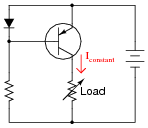If we were to crudely model the transistor’s behavior as an automatically-varied rheostat - constantly adjusting resistance as necessary to keep load current constant - how would you describe this rheostat’s response to changes in load resistance?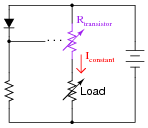In other words, as Rload increases, what does Rtransistor do - increase resistance, decrease resistance, or remain the same resistance it was before? How does the changing value of Rtransistor affect total circuit resistance?

• #### Question 5

Based on what you know about bipolar junction transistors, what will the collector current do (increase, decrease, or remain the same) if the variable voltage source increases in voltage? The small, fixed voltage source (0.7 volts) is just enough to make the transistor conduct, but not enough to fully saturate it.From the variable voltage source’s perspective, what does the transistor circuit “look” like? It certainly does not look resistive, because a resistive circuit would increase current linearly with increases in applied voltage! If you could relate the behavior of this circuit to a common idealized electrical component, what would it be?

• #### Question 6

Based on what you know about bipolar junction transistors, what will the collector current do (increase, decrease, or remain the same) if the variable resistor’s resistance is decreased? The small voltage source (0.7 volts) is just enough to make the transistor conduct, but not enough to fully saturate it.From the variable resistor’s perspective, what does the rest of the transistor circuit “look” like?

• #### Question 7

Describe what happens to the collector current of the transistor as the variable resistor’s value is changed: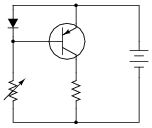Hint: it is helpful to remember that the voltage drop across a PN junction is not exactly constant as the current through it varies. There is a nonlinear relationship between diode voltage drop (VD) and diode current (ID) as described by the diode equation:

 $$I_D= I_S(e^{(\frac{qV_D}{NkT})} -1)$$

• #### Question 8

The circuit shown here is a simple current mirror. Explain what happens as the load resistance changes: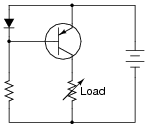Most current mirrors are not built exactly like this. Instead of a diode, they use a transistor (identical to the other transistor) with the base and collector terminals shorted together: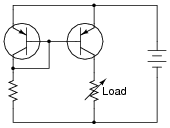Ideally, the two transistors are built on the same substrate material, so as to always be at equal temperature. Explain why this design is preferable to the first circuit (using the diode) shown in this question.

• #### Question 9

Two terms used commonly in electronics are sourcing and sinking, in reference to the direction of electric current between an active circuit and a load: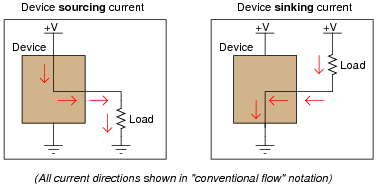A practical example of where this distinction is important is in certain integrated circuits (IC “chips”) where output pins may be able to only sink current, only source current, or both sink and source current.. Take a look at these two examples, each where an integrated circuit “chip” controls power to an LED. In one instance the IC is wired to source current to the LED, and in the other instance it is wired to sink current from the LED: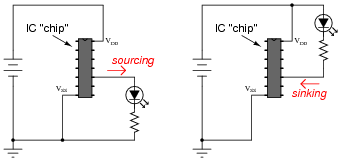If an IC is only able to do one or the other (source or sink current, but not both), it makes a big difference how you connect load devices to it! What makes the difference between a circuit that is able to source current versus a circuit that is able to sink current is the internal configuration of its transistors.

Similarly, a current mirror circuit may be built to either source current or sink current, but not do both. Draw current mirror circuits within the dotted-line boxes suitable for sourcing and sinking current to a load resistor: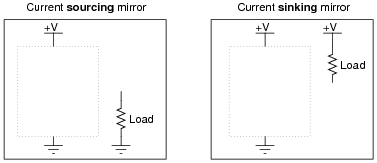• #### Question 10

Calculate the approximate amount of current this current mirror circuit will try to maintain through Rload, assuming silicon transistors (0.7 volts forward base-emitter junction drop):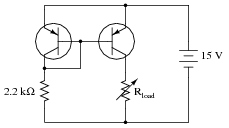• #### Question 11

Calculate the approximate amount of current this current mirror circuit will try to maintain through Rload, assuming silicon transistors (0.7 volts forward base-emitter junction drop):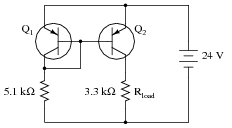Also, calculate the approximate power dissipation of transistor Q2.

• #### Question 12

Calculate the approximate amount of current this current mirror circuit will try to maintain through Rload, assuming silicon transistors (0.7 volts forward base-emitter junction drop):Also, calculate the approximate power dissipation of both transistors.

• #### Question 13

Choose a power supply voltage and resistance value for R1 that will maintain approximately 15 mA of current through the 1 kΩ load resistor. Assume the use of a silicon transistor: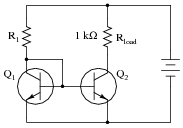To ensure plenty of regulation range (the ability to maintain regulated current over a wide range of load resistance values), design your circuit so that at least 20 volts VCE are dropped across transistor Q2. Also, calculate the approximate power dissipation of transistor Q2.

• #### Question 14

Describe the purpose of the transistor in this AC-DC power supply circuit: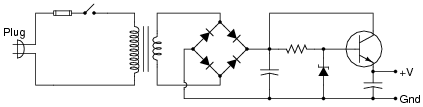• #### Question 15

Install a potentiometer in this circuit so that the regulated output voltage of this power supply becomes adjustable: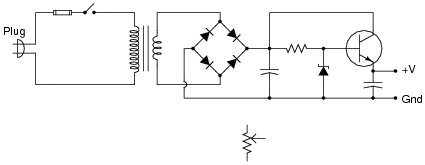Challenge: leave the potentiometer symbol in its place, and make the necessary wire connections between it and the rest of the circuit!

• #### Question 16

Calculate the approximate output voltage of this regulated power supply circuit and the amount of current through the zener diode under no-load conditions:• #### Question 17

Calculate the approximate output voltage of this regulated power supply circuit, the amount of current through the zener diode, and the (unregulated) voltage across the 1000 μF capacitor, all under no-load conditions: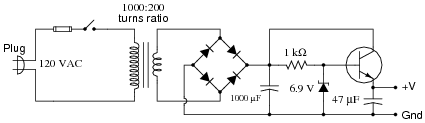• #### Question 18

Predict how all component voltages and currents in this circuit will be affected as a result of the following faults. Consider each fault independently (i.e. one at a time, no multiple faults):Resistor R1 fails open:
Transistor Q1 fails open, collector to emitter:
Transistor Q1 fails shorted, collector to emitter:
Transistor Q2 fails open, collector to emitter:
Transistor Q2 fails shorted, collector to emitter:

For each of these conditions, explain why the resulting effects will occur.

• #### Question 19

Predict how this power supply circuit’s output voltage will be affected as a result of the following faults. Also note whether or not any other components in this circuit will become stressed as a result of each fault. Consider each fault independently (i.e. one at a time, no multiple faults):Transformer T1 primary winding fails shorted:
Transformer T1 secondary winding fails open:
Rectifying diode D3 fails open:
Zener diode D5 fails open:
Zener diode D5 fails shorted:
Resistor R1 fails open:
Capacitor C2 fails shorted:

For each of these conditions, explain why the resulting effects will occur.

• #### Question 20

Suppose this regulated power supply circuit used to function fine, but now has stopped outputting any DC voltage at all: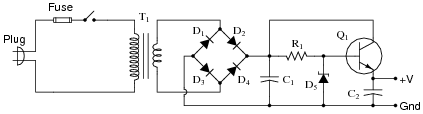Initial diagnostic measurements show there to be full DC (unregulated) voltage across capacitor C1, and no DC voltage between the transistor base and ground. From this data, where would you suspect the problem is?

• #### Question 21

Many different types of sensors work on the principle of a variable resistance representing a different physical quantity. One such sensor is the common fuel level sensor used in automotive, marine, and industrial fuel storage applications: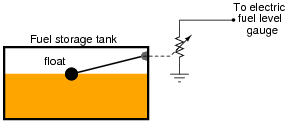As the fuel level in the tank changes, the float position will change, changing the sensor’s resistance. This resistance change is detected by an electric gauge (a special type of meter), which then provides visual indication of fuel level in the tank.

We must have some accurate way of measuring electrical resistance in order for this scheme to work. One common technique for doing this is to pass a constant current through the sensor resistance and then measure the voltage dropped across it. Since current mirror circuits function as current regulators, and therefore may be used as current sources if supplied with an external voltage, we could use a current mirror to force constant current through the fuel level sensor: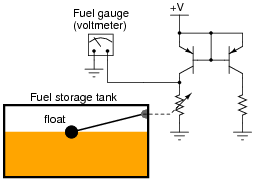One problem with the circuit shown is that the sensor current will change as the supply voltage ( V) changes. This may be important to us, because the DC system voltage on an automobile may not be very stable, and this could lead to inaccuracies in fuel level measurement.

Figure out a way we could use a zener diode to stabilize the voltage in this current mirror circuit so that supply voltage changes would have minimal effect on the amount of current through the variable-resistance sensor.

• #### Question 22

A very useful feature for a regulated voltage source is an electronic current limit: a circuit that limits the amount of current deliverable to a load, so as to avoid needless fuse-blowing. The combination of transistor Q2 and resistor R2 provides just this feature for the following voltage regulator circuit: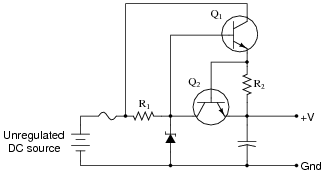Describe how transistor Q2 limits the current sourced to a direct short-circuit across the load terminals.

• #### Question 23

Suppose you had the boring job of manually maintaining the output voltage of a DC generator constant. Your one and only control over voltage is the setting of a rheostat: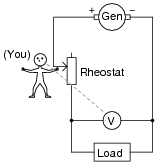What would you have to do to maintain the load voltage constant if the load resistance changed so as to draw more current? Being that your only control over load voltage is the adjustment of a variable resistance in series with the generator, what does this imply about the generator’s output voltage (directly across the generator terminals), compared to the target load voltage?

• #### Question 24

Suppose you had the boring job of manually maintaining the output voltage of a DC generator constant. Your one and only control over voltage is the setting of a rheostat: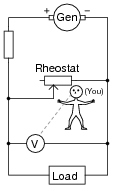What would you have to do to maintain the load voltage constant if the load resistance changed so as to draw more current? Being that your only control over load voltage is the adjustment of a variable resistance in parallel with the load, what does this imply about the generator’s output voltage (directly across the generator terminals), compared to the target load voltage?

• #### Question 25

Describe how a zener diode is able to maintain regulated (nearly constant) voltage across the load, despite changes in load current:• #### Question 26

Calculate the power dissipated by the 5-volt zener diode for the following values of motor current (assume the battery voltage remains constant at 12 volts):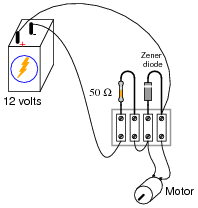Imotor = 20 mA ; Pzener =
Imotor = 50 mA ; Pzener =
Imotor = 90 mA ; Pzener =
Imotor = 120 mA ; Pzener =
Imotor = 150 mA ; Pzener =
• #### Question 27

Is it possible to reduce this zener diode voltage regulator circuit to a Thévenin equivalent circuit? Explain why or why not.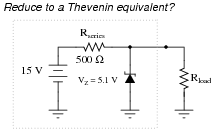• #### Question 28

Predict how all component voltages and currents in this circuit will be affected as a result of the following faults. Consider each fault independently (i.e. one at a time, no multiple faults):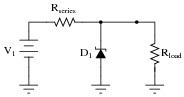Zener diode fails shorted:
Zener diode fails open:
Series resistor fails open:
Series resistor fails shorted:

For each of these conditions, explain why the resulting effects will occur.